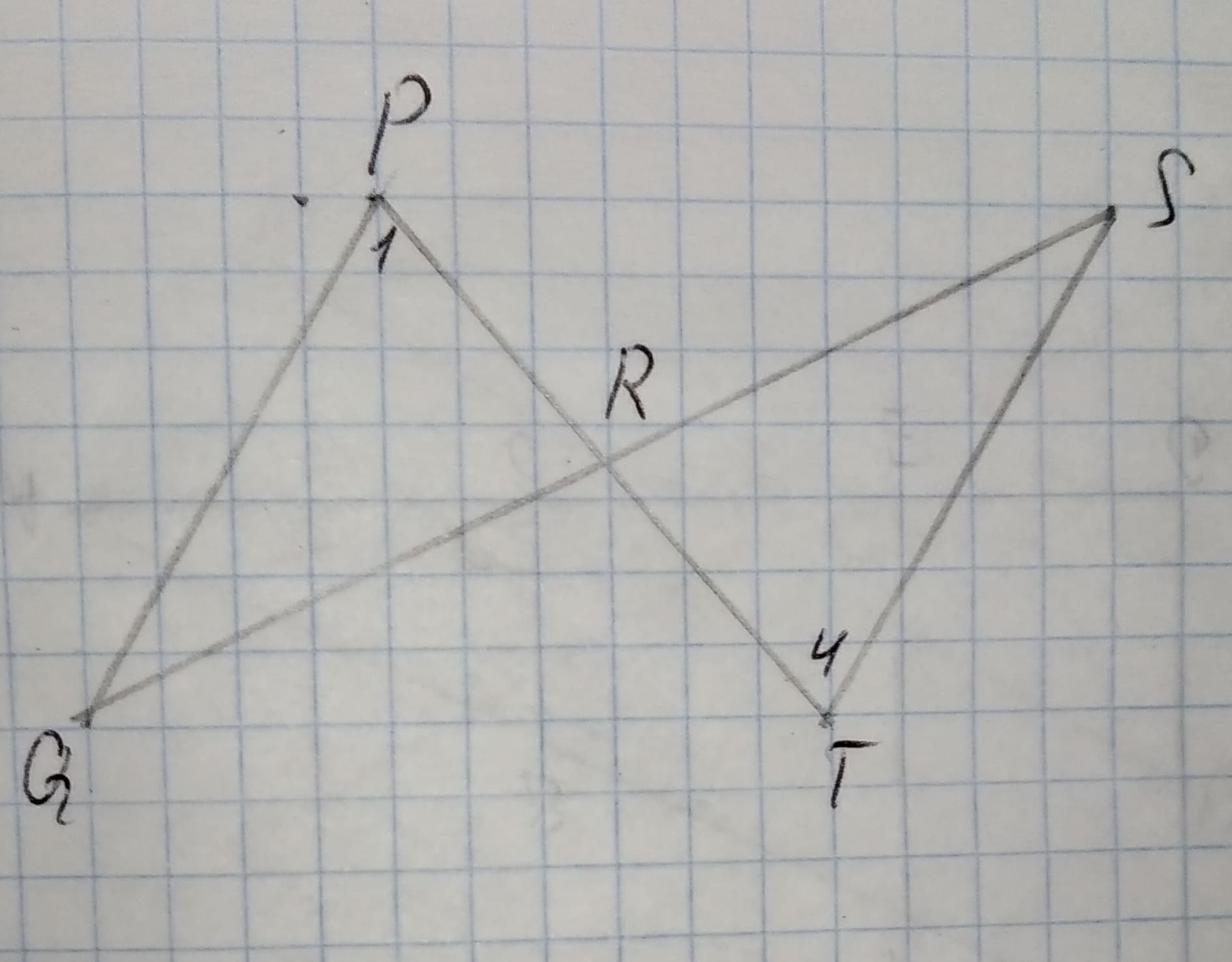# With of the following triangle congruence shortcuts could be used to prove PRQ = TRS12210203401.jpgGiven Data,/_Q ~= /_Sbar(QR) ~= bar(SR)a)Side-Side-Side Postulate (SSS)b)Side-Angle-Side Postulate (SAS)c)Angle-Side-Angle Postulate (ASA)d)Angle-Angle-Side Theorem (AAS)BenoguigoliB 2020-12-06 Answered

With of the following triangle congruence shortcuts could be used to prove PRQ = TRSGiven Data,
$\mathrm{\angle }Q\stackrel{\sim }{=}\mathrm{\angle }S$
$\stackrel{―}{QR}\stackrel{\sim }{=}\stackrel{―}{SR}$
a)Side-Side-Side Postulate (SSS)
b)Side-Angle-Side Postulate (SAS)
c)Angle-Side-Angle Postulate (ASA)
d)Angle-Angle-Side Theorem (AAS)

You can still ask an expert for help

## Want to know more about Congruence?

• Questions are typically answered in as fast as 30 minutes

Solve your problem for the price of one coffee

• Math expert for every subject
• Pay only if we can solve itEzra Herbert
Step 1
It is given that,
$\mathrm{\angle }Q\stackrel{\sim }{=}\mathrm{\angle }S$
$\stackrel{―}{QR}\stackrel{\sim }{=}\stackrel{―}{SR}$
$In\mathrm{△}PQR\phantom{\rule{1em}{0ex}}\text{and}\phantom{\rule{1em}{0ex}}\mathrm{△}RST$,
$\mathrm{\angle }Q\stackrel{\sim }{=}\mathrm{\angle }S$ (equal angles given)
$\stackrel{―}{QR}\stackrel{\sim }{=}\stackrel{―}{SR}$ ( equal sides given)
$\mathrm{\angle }PRQ\stackrel{\sim }{=}\mathrm{\angle }SRT$ ( vertically opposite angles)
Here, two angles and one side of both triangle is equal then,
Step 2
$\mathrm{△}PQR\stackrel{\sim }{=}\mathrm{△}RST$ (By angle-side-angle congruence)
Hence option C is correct.